# Notes 18 Expected Values Fair Game Play Expected

• Slides: 12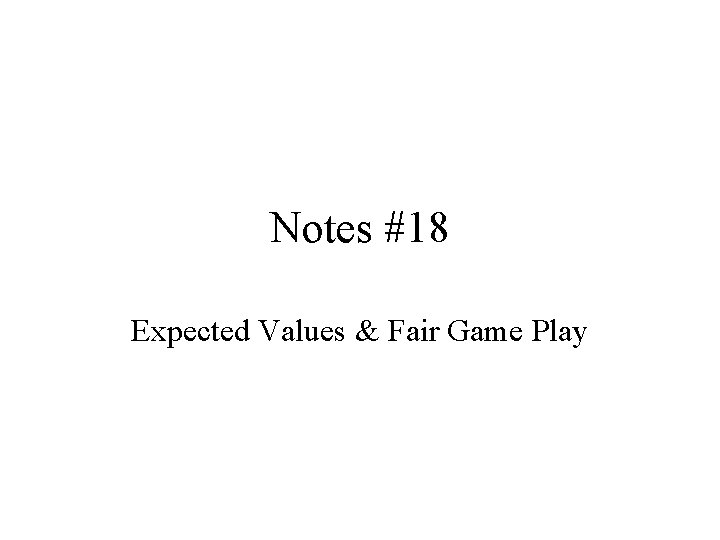Notes #18 Expected Values & Fair Game Play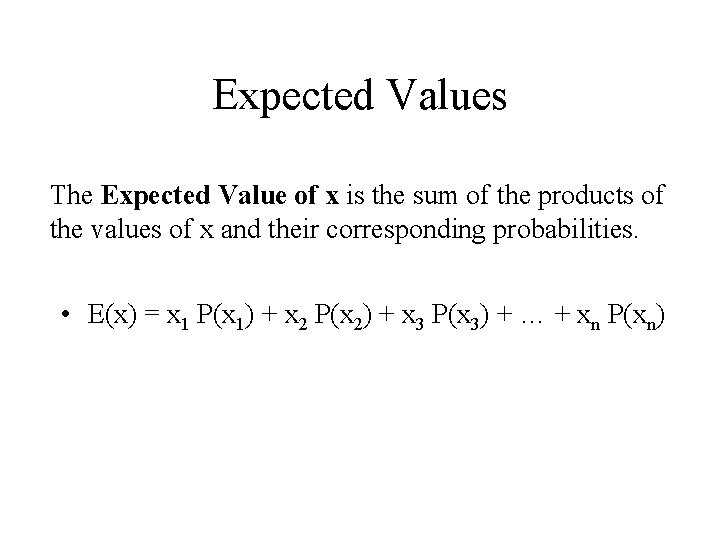Expected Values The Expected Value of x is the sum of the products of the values of x and their corresponding probabilities. • E(x) = x 1 P(x 1) + x 2 P(x 2) + x 3 P(x 3) + … + xn P(xn)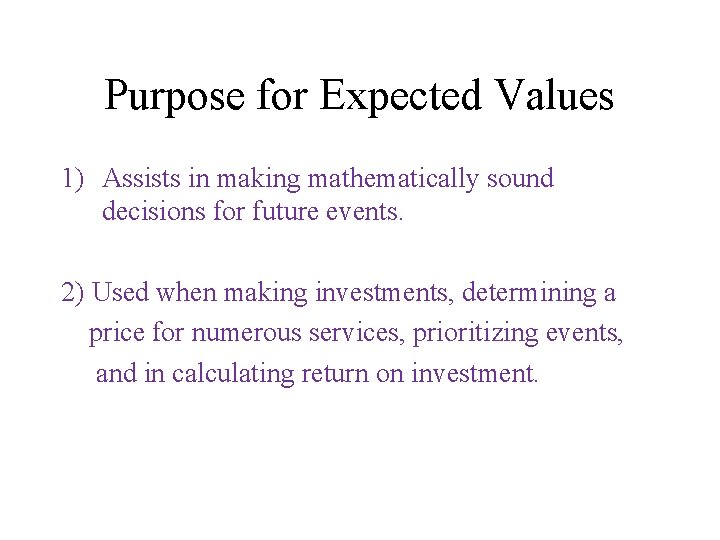Purpose for Expected Values 1) Assists in making mathematically sound decisions for future events. 2) Used when making investments, determining a price for numerous services, prioritizing events, and in calculating return on investment.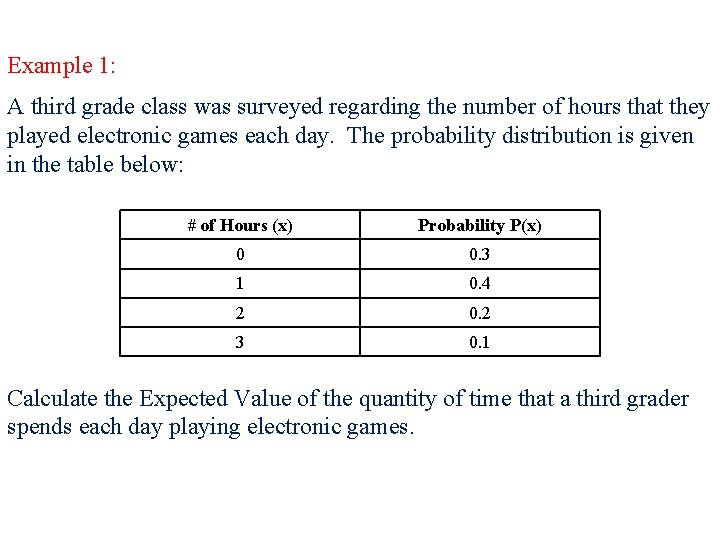Example 1: A third grade class was surveyed regarding the number of hours that they played electronic games each day. The probability distribution is given in the table below: # of Hours (x) Probability P(x) 0 0. 3 1 0. 4 2 0. 2 3 0. 1 Calculate the Expected Value of the quantity of time that a third grader spends each day playing electronic games.E(x) = x 1 P(x 1) + x 2 P(x 2) + x 3 P(x 3) + … + xn P(xn) # of Hours (x) Probability P(x) 0 0. 3 1 0. 4 2 0. 2 3 0. 1 Expected value, E(x) = 0 (0. 3) + 1 (0. 4) + 2 (0. 2) + 3 (0. 1) Expected value, E(x) = 0 + 0. 4 + 0. 3 Expected value, E(x) = 1. 1 hours Conclusion: Third graders spend 1. 1 hrs playing video games each day.Example 2: Find the expected number of boys for a three-child family. Assume girls and boys are equally likely. Key: b=Boy; g = Girl # of Boys Probability Product x P(x) bbg 0 1/8 0 bgb 1 3/8 2 3/8 6/8 3 1/8 3/8 Expected Value: E(x) =0 + 3/8 + 6/8 + 3/8 = 12/8 or 1. 5 boys 8 Combos bbb bgg gbb gbg ggb ggg Concl: The expected # of boys for a 3 -child family is 1. 5 boys.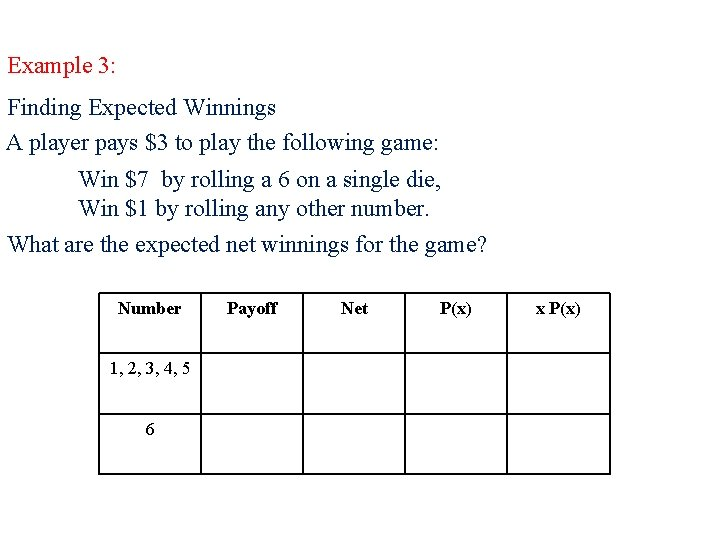Example 3: Finding Expected Winnings A player pays \$3 to play the following game: Win \$7 by rolling a 6 on a single die, Win \$1 by rolling any other number. What are the expected net winnings for the game? Number 1, 2, 3, 4, 5 6 Payoff Net P(x) x P(x)Finding Expected Winnings A player pays \$3 to play the following game: Win \$7 by rolling a 6 on a single die, Win \$1 by rolling any other number. What are the expected net winnings for the game? ANS: The player will not have an expected net winning for the game, since his Expected Value is a loss of \$1. 00.Fair Games/Expected Value • The expected value of a game is the amount, on average, of money you win per game. The expected value (in terms of a game) is calculated as follows: • E = (\$ paid if you win) * (P(winning)) • A game is a fair game when the cost of each game equals the expected value (what you put in, you get out).A fair game is one in which the net winnings are zero. An unfair game against the player has negative expected winnings. An unfair game in favor of the player has positive expected winnings.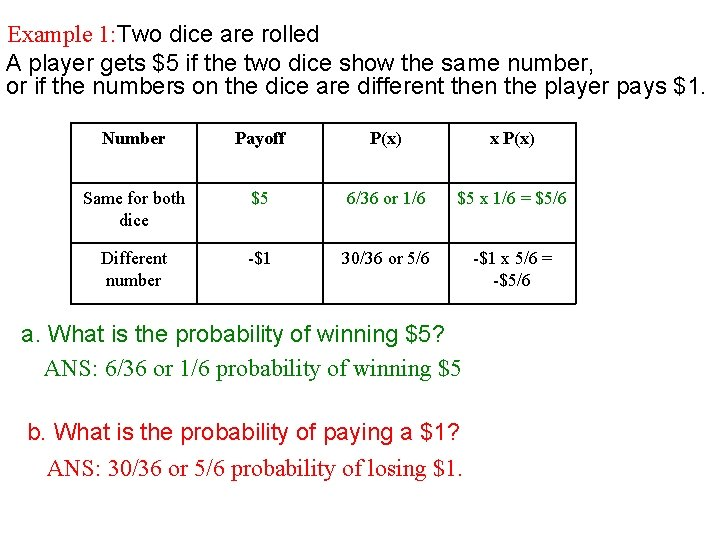Example 1: Two dice are rolled A player gets \$5 if the two dice show the same number, or if the numbers on the dice are different then the player pays \$1. Number Payoff P(x) x P(x) Same for both dice \$5 6/36 or 1/6 \$5 x 1/6 = \$5/6 Different number -\$1 30/36 or 5/6 -\$1 x 5/6 = -\$5/6 a. What is the probability of winning \$5? ANS: 6/36 or 1/6 probability of winning \$5 b. What is the probability of paying a \$1? ANS: 30/36 or 5/6 probability of losing \$1.Example 1: A player gets \$5 if the two dice show the same number, or if the numbers on the dice are different then the player pays \$1. Number Payoff P(x) x P(x) Same for both dice \$5 6/36 or 1/6 \$5 x 1/6 = \$5/6 Different number -\$1 30/36 or 5/6 -\$1 x 5/6 = \$5/6 c. What is the expected value of this game? E(x) = x 1 P(x 1) + x 2 P(x 2) + x 3 P(x 3) + … + xn P(xn) E(x) = \$5(1/6) + (-\$1)(5/6) = \$5/6 – \$5/6 = 0 The Expectation is \$0. This would be a fair game.